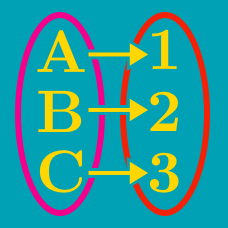Probability

# Definition of Bijection, Injection, and Surjection

$15$ football teams are competing in a knock-out tournament. Each game has a winner, there are no draws, and the losing team is out of the tournament. How many games need to be played in order for a tournament champion to be determined?

For two sets $X=\{-1,1,a\}, Y=\{8,9, b\},$ $f(x)=x^3+9$ is a bijective function from $X$ to $Y$. What is the value of $a+b$?

For the two sets $X=\{a, b, c\}$ and $Y=\{9, 17, 72\},$ how many bijective functions are possible from $X$ to $Y$?

Suppose $f(x)=ax+3$ is a bijective function from $\mathbb{R}$ to $\mathbb{R}$. If $f(7)=38,$ what value of $x$ satisfies $f(x) =103?$

Suppose $f(x)=ax+3$ is a bijective function from $\mathbb{R}$ to $\mathbb{R}$. If $f(5)=28,$ what value of $x$ satisfies $f(x) =78?$

×